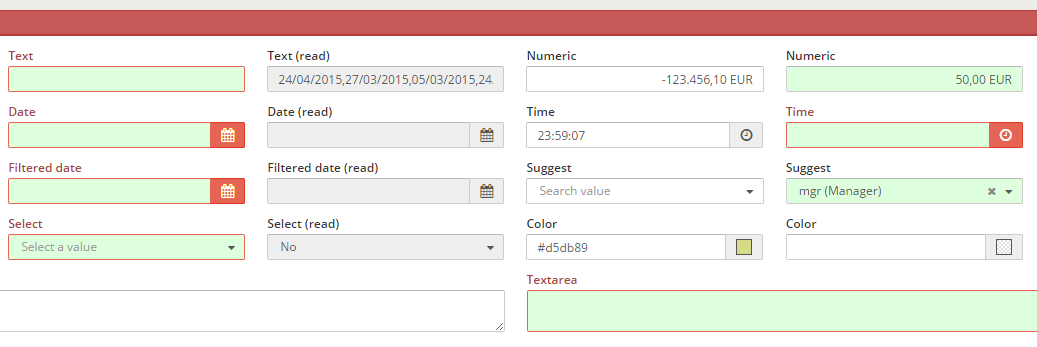Version: 4.4.0

Validation

The validation action checks the conditions of each criterion with a value under the validation attribute. This action is related with a validate type button (see button actions).XML skeleton​

<criteria [attributes] validation="[condition to validate]" />

Validation types​

There are some kind of validation:

Value of attributeComponentDescriptionExamples
requiredAll except checkbox and radioCheck if the field has valuevalidation="{required:true}"
textText / Textarea / PasswordCheck if the value of the criteria is a text (Numbers and white spaces are not allowed)validation="{text:true}"
textWithSpacesText / Textarea / PasswordCheck if the value of the criteria is a text (Numbers not allowed)validation="{textWithSpaces:true}"
numberText / Textarea / PasswordCheck if the value of the criteria is a numbervalidation="number"
integerText / Textarea / PasswordCheck if the value is a well-formed integervalidation="integer"
digitsText / Textarea / PasswordCheck if the value is a number with only digitsvalidation="digits"
dateText / Textarea / PasswordCheck if the value is a valid datevalidation="date"
timeText / Textarea / PasswordCheck if the value is a valid time (hour, minutes and seconds)validation="time"
emailText / Textarea / PasswordCheck if the value is a valid string emailvalidation="email"
gtAll except checkbox and radioCheck if the criterion value is greater than valuevalidation="{gt:value}"
geAll except checkbox and radioCheck if the criterion value is greater or equal valuevalidation="{ge:value}"
ltAll except checkbox and radioCheck if the criterion value is lower than valuevalidation="{lt:value}"
leAll except checkbox and radioCheck if the criterion value is lower or equal than valuevalidation="{le:value}"
eqAll except checkbox and radioCheck if the criterion value is equal than valuevalidation="{eq:value}"
neAll except checkbox and radioCheck if the criterion value is different than valuevalidation="{ne:value}"
modNumeric / Date / TimeCheck if the criterion value is divisible by valuevalidation="{mod:value}"
rangeNumeric / Date / TimeCheck if the value is inside the rangevalidation="{range:{from:value, to:value}}"
equallengthText / Textarea / PasswordCheck if the text length is equal to valuevalidation="{equallegth:value}"
maxlengthText / Textarea / PasswordCheck if the text length is less than valuevalidation="{maxlegth:value}"
minlengthText / Textarea / PasswordCheck if the text length is bigger than valuevalidation="{minlength:value}"
checkAtLeastCheckbox / Button checkboxAssure that there is at least value checked checkboxes in the groupvalidation="{checkAtLeast:value}"
patternText / Textarea / PasswordCheck if the text is equal to the parametervalidation="{pattern:value}"

Validation values​

You can assign a simple static value to the validation comparator:

{eq:3}

... or you can take the value from a setting or a criterion:

{eq:{criterion:"CriterionId",type:"date"}}

These are the possible attributes on a value comparator:

Value of attributeDescriptionExamples
valueConstant value to compare withvalue:5
criterionRetrieve the value from a criterioncriterion:"CriterionId"
settingRetrieve the value from a settingsetting:"minlengthPassword"
messageLocale from message to retrieve if validation is not passedmessage:"VALIDATION_MESSAGE_CUSTOM_ERROR"
typeType of comparisontype:"type"

Comparison types​

You can define the type of the comparison, to check the values as a defined type:

TypeDescription
dateCompare as dates
integerCompare as integer
floatCompare as floats
stringCompare as strings (default)

Multiple validation​

It is possible to have more than one validation actions under a single validation attribute. To do so, it is necessary to insert the validations in a json format:

{eq:{criterion:"Field1"},ne:{criterion:"Field2"}}

There is an exception with simple validation rules that have no parameters, such as required. In this case, it's not necessary to have the rule between {} brackets:

validation="required"

If you want to combine simple rules with complex rules, you need to define it as a complex rule as well:

{required:true, maxlength:{value:6, type:"integer"}}

Examples​

Standard criteria with validation action​

<criteria label="PARAMETER_TEXT" id="TxtReq" variable="TxtReq" component="text" style="col-xs-6 col-sm-3 col-lg-2" validation="{required:true, maxlength:4}"/>

Check that the password is valid​

<criteria label="PARAMETER_PASSWD" id="Pas" component="text"